# Fused hard sphere chains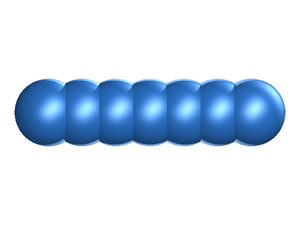Example of the fused hard sphere chain model, shown here in a linear configuration.

In the fused hard sphere chain model the molecule is built up form a string of overlapping hard sphere sites, each of diameter$\sigma$.

An effective number of monomers can be applied to the fused hard sphere chain model by using the relarion (Ref.  Eq. 2.18)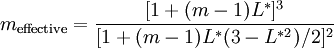$m_{\rm effective} = \frac{[1+(m-1)L^*]^3}{[1+(m-1)L^*(3-L^{*2})/2]^2}$

where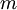$m$ is the number of monomer units in the model, and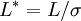$L^*=L/\sigma$ is the reduced bond length.

The volume of the fused hard sphere chain is given by (Ref.  Eq. 13)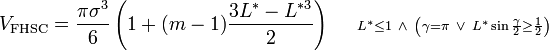$V_{\rm FHSC} =\frac{\pi \sigma^3}{6} \left( 1 + (m-1)\frac{3L^* - L^{*3}}{2} \right) ~~~~ \scriptstyle{ L^* \leq 1 ~\and~ \left(\gamma=\pi ~ \or ~ L^* \sin{\frac\gamma{2}} \geq \frac{1}{2}\right) }$

where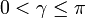$0<\gamma \leq \pi$ is the minimal bond angle, and the surface area is given by (Ref. Eq. 12)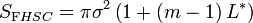$S_{\mathrm FHSC} = \pi \sigma^2 \left( 1+\left( m-1 \right) L^* \right)$

## Equation of state

The Vörtler and Nezbeda equation of state is given by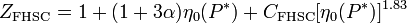$Z_{\mathrm{FHSC}}= 1+ (1+3\alpha)\eta_0(P^*) + C_{\rm FHSC}[\eta_0(P^*)]^{1.83}$

where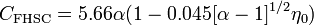$C_{\rm FHSC} = 5.66\alpha(1-0.045[\alpha-1]^{1/2}\eta_0)$

and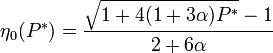$\eta_0(P^*) = \frac{\sqrt{1+4(1+3\alpha)P^*}-1}{2+6\alpha}$

The Waziri and Hamad equation of state for fused hard sphere chain fluids is given by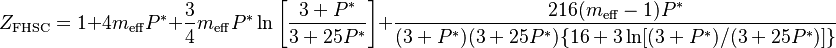$Z_{\mathrm{FHSC}} = 1 + 4m_{\mathrm{eff}}P^{*} + \frac{3}{4}m_{\mathrm{eff}}P^{*}\ln\left[\frac{3+P^{*}}{3+25P^{*}}\right] + \frac{216(m_{\mathrm{eff}} - 1)P^{*}}{(3+P^{*})(3+25P^{*})\{16+3\ln[(3+P^{*})/(3+25P^{*})]\}}$

where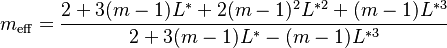$m_{\mathrm{eff}}=\frac{2+3(m-1)L^{*}+2(m-1)^{2}L^{*2}+(m-1)L^{*3}}{2+3(m-1)L^{*}-(m-1)L^{*3}}$